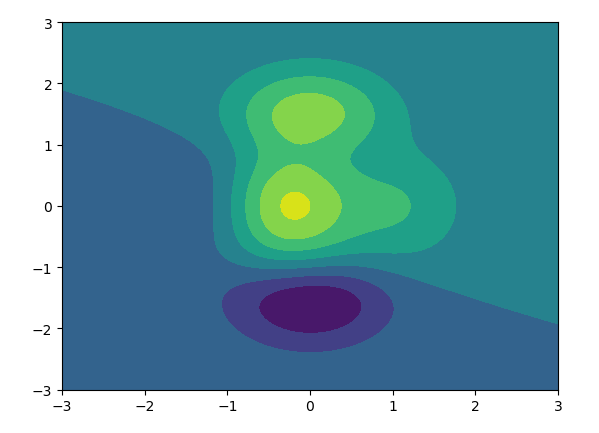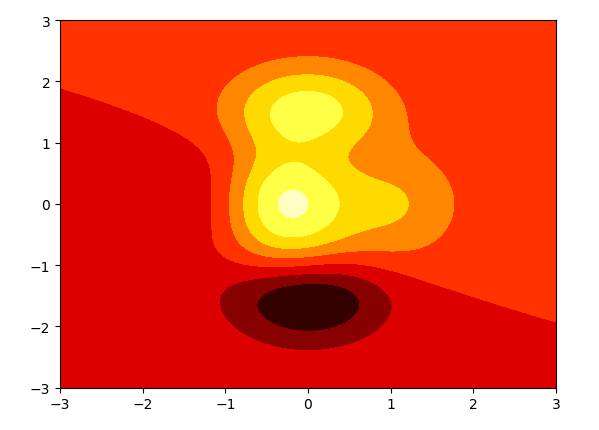matplotlib.pyplot contourf

coutour([X, Y,] Z,[levels], **kwargs)

 引數： X,Y：類似陣列，可選 為Z中的座標值 當 X,Y,Z 都是 2 維陣列時，它們的形狀必須相同。如果都是 1 維陣列時，len(X)是 Z 的列數，而 len(Y) 是 Z 中的行數。（例如，經由建立numpy.meshgrid()） Z：類似矩陣 繪製輪廓的高度值 levels：int或類似陣列，可選 確定輪廓線/區域的數量和位置 其他引數： aalpha：float ，可選 alpha混合值，介於0（透明）和1（不透明）之間。 cmap：str或colormap ，可選 Colormap用於將資料值（浮點數）從間隔轉換為相應Colormap表示的RGBA顏色。用於將資料縮放到間隔中看 。

Example：

import numpy as np
import pandas as pd
import matplotlib.pyplot as plt

# 計算x,y座標對應的高度值
def f(x, y):
return (1-x/2+x**3+y**5) * np.exp(-x**2-y**2)

# 生成x,y的資料
n = 256
x = np.linspace(-3, 3, n)
y = np.linspace(-3, 3, n)

# 把x,y資料生成mesh網格狀的資料，因為等高線的顯示是在網格的基礎上新增上高度值
X, Y = np.meshgrid(x, y)

# 填充等高線
plt.contourf(X, Y, f(X, Y))
# 顯示圖表
plt.show()# 填充等高線
plt.contourf(X, Y, f(X, Y), cmap=plt.cm.hot)